## Selina Concise Mathematics Class 10 ICSE Solutions Chapter 20 Cylinder, Cone and Sphere (Surface Area and Volume) Ex 20G

These Solutions are part of Selina Concise Mathematics Class 10 ICSE Solutions. Here we have given Selina Concise Mathematics Class 10 ICSE Solutions Chapter 21 Trigonometrical Identities Ex 20G.

Other Exercises

Question 1.
What is the least number of solid metallic spheres, each of 6 cm diameter, that should be melted and recast to form a solid metal cone whose height is 45 cm and diameter 12 cm ?
Solution:
Diameter of cone = 12cm
∴ Radius (r1) = $$\frac { 12 }{ 2 }$$= 6cm
Height (A) = 45 cm
∴  Volume = $$\frac { 1 }{ 3 }$$πR2h
= $$\frac { 1 }{ 3 }$$ x π x 6 x 6 x 45 cm3
= 540 π cm2
∴ Volume of solid spheres = 540 π cm3
Diameter of one sphere = 6cm
∴  Radius = $$\frac { 6 }{ 2 }$$ = 3 cm
∴ Volume of one spherical ballQuestion 2.
A largest sphere is to be carved out of a right circular cylinder of radius 7 cm and height 14 cm. Find the volume of the sphere. (Answer correct to the nearest integer).
Solution:
Radius of cylinder = 7 cm
and height 14 cm
By carving a largest sphere from it,
the radius of the sphere will be = 7 cm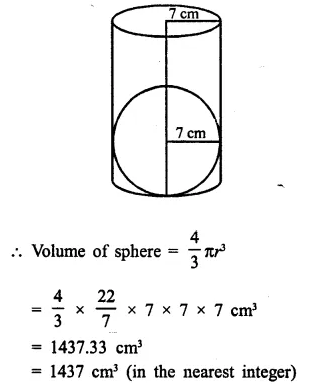Question 3.
A right circular cylinder having diameter 12 cm and height 15 cm is full of ice-cream. The ice-cream is to be filled in identical cones of height 12 cm and diameter 6 cm having a hemi­spherical shape on the top. Find the number of cones  required.
Solution:
Diameter of cylinder = 12 cm
∴ Radius (r) = $$\frac { 12 }{ 2 }$$ = 6 cm
Height (h) = 15 cm
Volume of ice-cream in cylinder = πr²h = π x 6 x 6 x 15 = 540π cm3
∴  Volume of ice-cream = 540π cm3
Now diameter of cone = 6 cmQuestion 4.
A solid is in the form of a cone standing on a hemi-sphere with both their radii being equal to 8 cm and the height of cone is equal to its radius. Find, in terms of π , the volume of the solid.
Solution:
Radius of each cone and hemi-sphere (r) = 8 cm
Height of cone (h) = r = 8 cm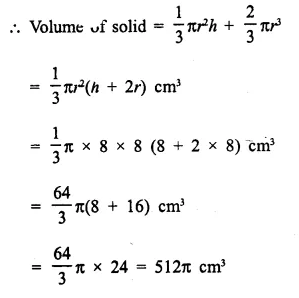Question 5.
The diameter of sphere is 6 It is melted and drawn into a wire of diameter 0.2 cm. Find the length of the wire.
Solution:
Diameter of a sphere = 6 cm.
∴
Radius = $$\frac { 6 }{ 2 }$$ = 3 cm.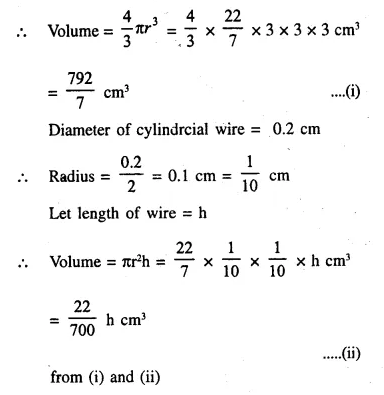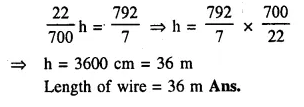Question 6.
Determine the ratio of the volume of a cube to that of a sphere which will exactly fit inside the cube.
Solution:
Let edge of the cube = a
∴ Volume of cube = a x a x a = a3
The sphere, which exactly fits in the cube, has radius = $$\frac { a }{ 2 }$$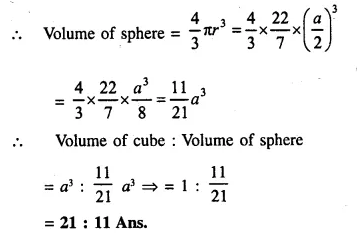Question 7.
An iron pole consisting of a cylindrical por­tion 110 cm high and of base diameter 12 cm is surmounted by a cone 9 cm high. Find the mass of the pole, given that 1cm3 of iron has 8 gm of mass (approx).Solution:
Radius of the base of poles (r) = $$\frac { 12 }{ 2 }$$ = 6cm.P.Q.
When a metal cube is completely submerged in water contained in a cylindrical vessel with diameter 30 cm, the level of water rises by 1 $$\frac { 41 }{ 99 }$$ cm. Find:
(i) the length of the edge of the cube.
(ii) the total surface area of the cube.
Solution:
Diameter of cylinderical vessel = 30 cm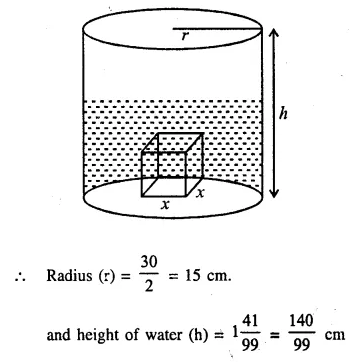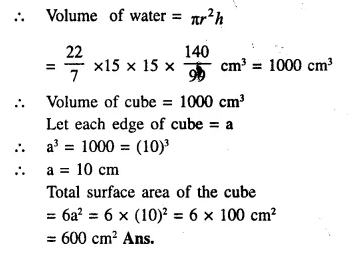Question 8.
In the following diagram a rectangular plat­form with a semi-circular end on one side is 22 metres long from one end to the other end. If the length of the half circumference is 11 metres, find the cost of constructing the platform, 1.5 metres high at the rate of Rs. 4 per cubic metres.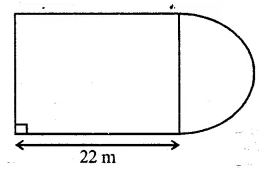Solution:
Length of platform = 22m
Circumference of semicircle = 11m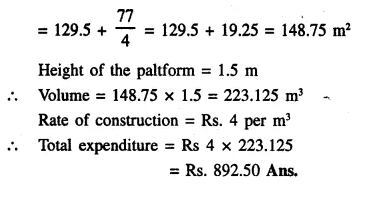Question 9.
The cross-section of a tunnel is a square of side 7 m surmounted by a semi-circle as shown in the following figure. The tunnel is 80 m long. Calculate :
(i) Its volume
(ii) The surface area of the tunnel (excluding the floor) and
(iii) its floor area.Solution:
Side of square = 7m
Radius of semicircle = $$\frac { 7 }{ 2 }$$ m
Length of tunnel = 80 m.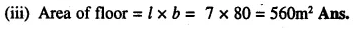Question 10.
A cylindrical water tank of diameter 2.8 m and height 4.2 m is being fed by a pipe of diam­eter 7 cm through which water flows at the rate of 4 ms-1. Calculate, in minutes, the time it takes to fill the tank.
Solution:
Diameter of cylindrical tank = 2.8 m 2.8
∴ Radius (r) = $$\frac { 2.8 }{ 2 }$$ = 1.4 m
and height (h) = 4.2 m
Volume of water filled in it = πr2h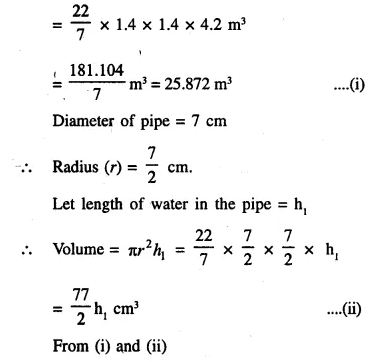Question 11.
Water flows, at 9 km per hour, through a cylindrical pipe of cross-sectional area 25 cm2. If this water is collected into a rectangular cistern of dimensions 7.5 m by 5 m by 4 m; calculate the rise in level in the cistern in 1 hour 15 minutes.
Solution:
Rate of flow of water = 9km / hr
∴ Water flow in 1 hr 15 minutes i.e. in $$\frac { 5 }{ 4 }$$  hrQuestion 12.
The given figure shows the cross-section of a cone, a cylinder and a hemisphere all with the same diameter 10 cm, and the other dimensions are as shown. Calculate :
(a) the total surface area,
(b) the total volume of the solid and
(c) the density of the material if its total weight is 1.7 kg.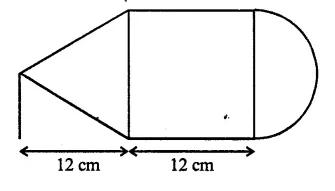Solution:
Diameter = 10 cm
∴  Radius (r) =  $$\frac { 10 }{ 2 }$$   = 5 cm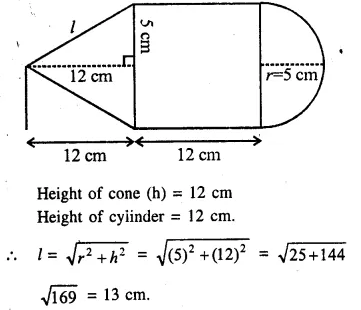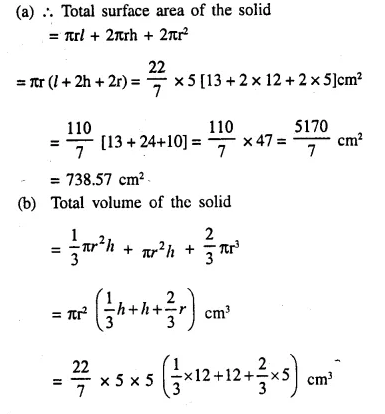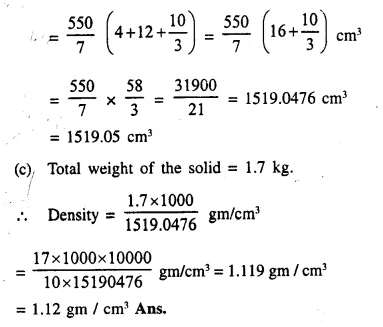Question 13.
A solid, consisting of a right circular cone, standing on a hemisphere, is placed upright, in a right circular cylinder, full of water, and touches the bottom. Find the volume of water left in the cylinder, having given that the radius of the cylinder is 3 cm and its height is 6 cm; the radius of the hemisphere is 2 cm and the height of cone is 4 cm. Give your answer to the nearest cubic centimetre. 
Solution:
Radius of cylinder = 3 cm
and height = 6 cm
Radius of hemisphere = 2 cm
and height of cone = 4 cm

Volume of water in the cylinder when it is full
= πr2h = π x (3)2 x 6 cm3 = 54π cm3
Volume of water displaced = Volume of cone + volume of hemisphere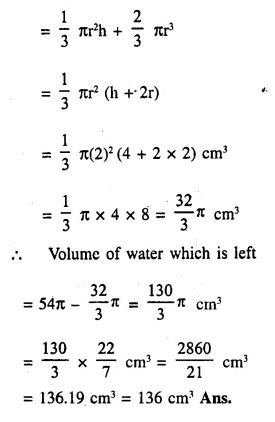Question 14.
A metal container in the form of a cylinder is surmounted by a hemi-sphere of the same radius. The internal height of the cylinder is 7 m and the internal radius is 3.5 m. Calculate
(i) he total area of the internal surface, exclud­ing the base;
(ii) the internal volume of the container in m3 
Solution:
Radius of cylinder = 3.5 m
and height = 7 m.
(i) Total surface area of container excluding the base = curved surface area of cylinder + area of hemisphere = 2πrh + 2πr2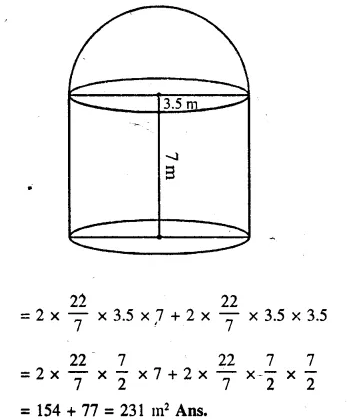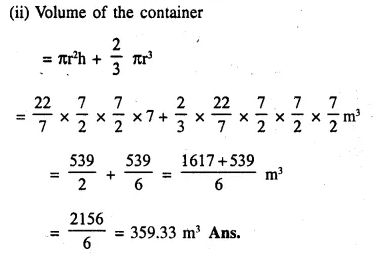Question 15.
An exhibition tent is in the form of a cylin­der surmounted by a cone. The height of the tent above the ground is 85 m and height of the cylindrical part is 50 m. If the diameter of the base is 168 m, find the quantity of canvas required to make the tent. Allow 20% extra for fold and for stitching. Give your answer to the nearest m2.     
Solution:
Total height of the tent = 85 m.
Daimeter of the base = 168 m.
∴ Radius (r) = $$\frac { 168 }{ 2 }$$ = 84 m
Height of cylindrical part = 50 m
Then height of conical part (h) = 85 – 50 = 35 m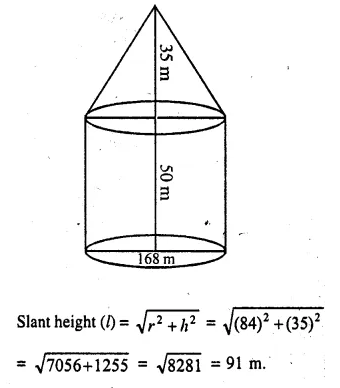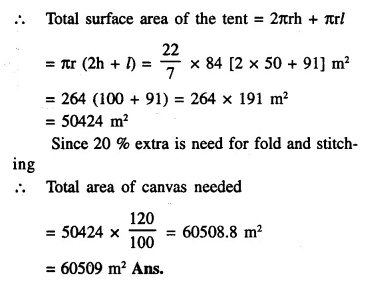P.Q.
The total surface area of a hollow cylinder, which is open from both sides is 3575 cm2; area of the base ring is 357.5 cm2 and height is 14 cm. Find the thickness of the cylinder.
Solution:
Total surface area of a hollow cylinder = 3575 cm2
Area of base ring = 357.5 cm2
Height = 14 cm.
and let thickness of cylinder = (R – r) = d
∴  Total surface area = 2πRh + 2πrh + 2π (R2– r2)
= 2πh (R + r) + 2π (R + r) (R – r)
= 2π (R + r) [h + R – r]
= 2π (R + r) (h + d)
= 2π (R + r) (14 + d)
But 2π (R + r) (14 + d) = 3575                  ….(i)
and area of the base = π (R2 – r2) = 357.5
⇒  π (R + r) ( R – r) = 357.5
⇒ π (R + r)d = 357.5                                 ….(ii)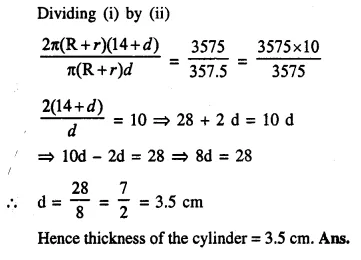Question 16.
A test tube consists of a hemisphere and a cylinder of the same radius. The volume of the water required to fill the whole tube  is $$\frac { 5159 }{ 6 }$$cm3, and $$\frac { 4235 }{ 6 }$$ cm3 of water is required to fill the tube to a level which is 4 cm below the top of the tube. Find the radius of the tube and the length of its cylindrical part.
Solution: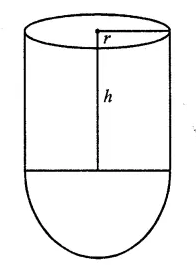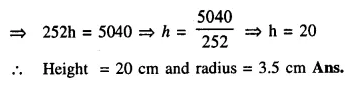Question 17.
A solid is in the form of a right circular cone mounted on a hemisphere. The diameter of the base of the cone, which exactly coincides with hemisphere, is 7cm and its height is 8cm. The solid is placed in a cylindrical vessel of internal radius 7 cm and height 10cm. How much water, in cm3, will be required to fill the vessel completely.
Solution:
Diameter of hemisphere = 7cm
Diameter of the base of a cone = 7cm
∴ Radius (r) = $$\frac { 7 }{ 2 }$$ cm = 3.5 cm
Height (h) = 8cm.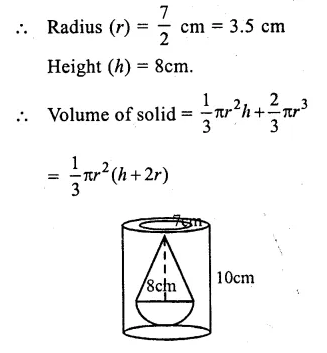Question 18.
Two solid spheres of radii 2 cm and 4 cm are melted and recast into a cone of height 8 cm. Find the radius of the cone so formed.   (2015)
Solution:
Radius of first sphere = 2 cmQuestion 19.
A certain number of metallic cones, each of radius 2 cm and height 3 cm are melted and recast into a solid sphere of radius 6 cm. Find the number of cones used.             (2016)
Solution:
Let the number of cones be n,
Let radius of the sphere be rs, radius of a cone be rc and h be the height of the cone.
Volume of sphere = n(Volume of a metallic cone)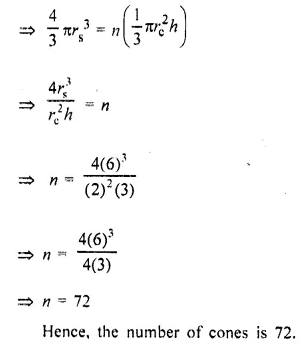Hope given Selina Concise Mathematics Class 10 ICSE Solutions Chapter 20 Cylinder, Cone and Sphere Ex 20G are helpful to complete your math homework.

If you have any doubts, please comment below. Learn Insta try to provide online math tutoring for you.